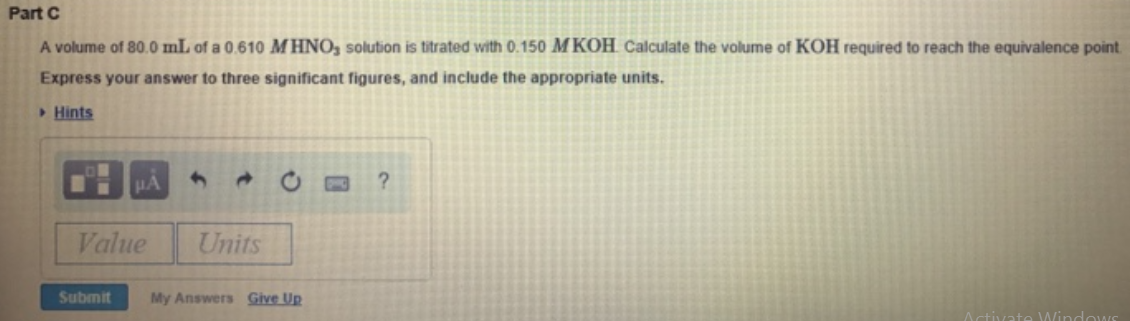# Problem: A volume of 80.0 mL of a 0.610 M HNO3 solution is titrated with 0.150 M KOH. Calculate the volume of KOH required to reach the equivalence point. Express your answer to three significant figures, and include the appropriate units.

###### FREE Expert Solution
92% (497 ratings)###### Problem Details

A volume of 80.0 mL of a 0.610 M HNO3 solution is titrated with 0.150 M KOH. Calculate the volume of KOH required to reach the equivalence point.

Express your answer to three significant figures, and include the appropriate units.